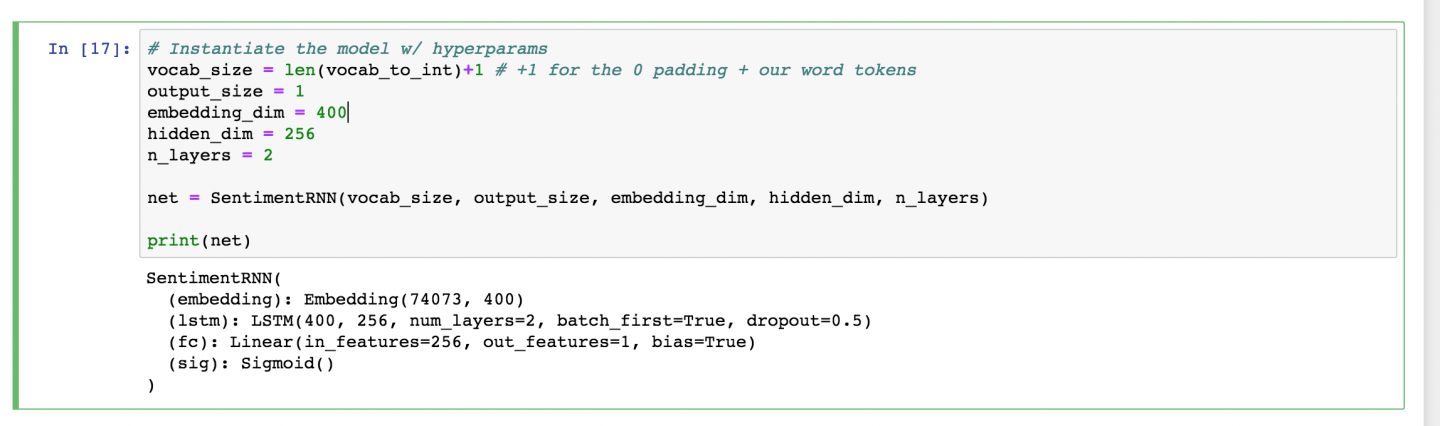# AI For Trading: Training the Model (102)

## Hyperparameters

After defining my model, next I should instantiate it with some hyperparameters.

# Instantiate the model w/ hyperparams
vocab_size = len(vocab_to_int)+1 # +1 for the 0 padding + our word tokens
output_size = 1
embedding_dim = 400
hidden_dim = 256
n_layers = 2

net = SentimentRNN(vocab_size, output_size, embedding_dim, hidden_dim, n_layers)

print(net)

This should look familiar, but the main thing to note here is our vocab_size.

This is actually the length of our vocab_to_int dictionary (all our unique words)plus one to account for the 0-token that we added, when we padded our input features. So, if you do data pre-processing, you may end up with one or two extra, special tokens that you’ll need to account for, in this parameter!

Then, I want my output_size to be 1; this will be a sigmoid value between 0 and 1, indicating whether a review is positive or negative.

Then I have my embedding and hidden dimension. The embedding dimension is just a smaller representation of my vocabulary of 70k words and I think any value between like 200 and 500 or so would work, here. I’ve chosen 400. Similarly, for our hidden dimension, I think 256 hidden features should be enough to distinguish between positive and negative reviews.

I’m also choosing to make a 2 layer LSTM. Finally, I’m instantiating my model and printing it out to make sure everything looks good.Model hyperparameters

## Training and Optimization

The training code, should look pretty familiar. One new detail is that, we'll be using a new kind of cross entropy loss that is designed to work with a single Sigmoid output.

BCELoss, or Binary Cross Entropy Loss, applies cross entropy loss to a single value between 0 and 1.

We'll define an Adam optimizer, as usual.

# loss and optimization functions
lr=0.001

criterion = nn.BCELoss()
optimizer = torch.optim.Adam(net.parameters(), lr=lr)

## Output, target format

You should also notice that, in the training loop, we are making sure that our outputs are squeezed so that they do not have an empty dimension output.squeeze() and the labels are float tensors, labels.float(). Then we perform backpropagation as usual.

## Train and eval mode

Below, you can also see that we switch between train and evaluation mode when the model is training versus when it is being evaluated on validation data!

## Training Loop

Below, you’ll see a usual training loop.

I’m actually only going to do four epochs of training because that's about when I noticed the validation loss stop decreasing.

• You can see that I am initializing my hidden state before entering the batch loop then have my usual detachment from history for the hidden state and backpropagation steps.
• I’m getting my input and label data from my train_dataloader. Then applying my model to the inputs and comparing the outputs and the true labels.
• I also have some code that checks performance on my validation set, which, if you want, may be a great thing to use to decide when to stop training or which best model to save!
# training params

epochs = 4 # 3-4 is approx where I noticed the validation loss stop decreasing

counter = 0
print_every = 100

# move model to GPU, if available
if(train_on_gpu):
net.cuda()

net.train()
# train for some number of epochs
for e in range(epochs):
# initialize hidden state
h = net.init_hidden(batch_size)

# batch loop
counter += 1

if(train_on_gpu):
inputs, labels = inputs.cuda(), labels.cuda()

# Creating new variables for the hidden state, otherwise
# we'd backprop through the entire training history
h = tuple([each.data for each in h])

# get the output from the model
output, h = net(inputs, h)

# calculate the loss and perform backprop
loss = criterion(output.squeeze(), labels.float())
loss.backward()
# clip_grad_norm helps prevent the exploding gradient problem in RNNs / LSTMs.
optimizer.step()

# loss stats
if counter % print_every == 0:
# Get validation loss
val_h = net.init_hidden(batch_size)
val_losses = []
net.eval()

# Creating new variables for the hidden state, otherwise
# we'd backprop through the entire training history
val_h = tuple([each.data for each in val_h])

if(train_on_gpu):
inputs, labels = inputs.cuda(), labels.cuda()

output, val_h = net(inputs, val_h)
val_loss = criterion(output.squeeze(), labels.float())

val_losses.append(val_loss.item())

net.train()
print("Epoch: {}/{}...".format(e+1, epochs),
"Step: {}...".format(counter),
"Loss: {:.6f}...".format(loss.item()),
"Val Loss: {:.6f}".format(np.mean(val_losses)))

Make sure to take a look at how training and validation loss decrease during training! Then, once you're satisfied with your trained model, you can test it out in a couple ways to see how it behaves on new data!